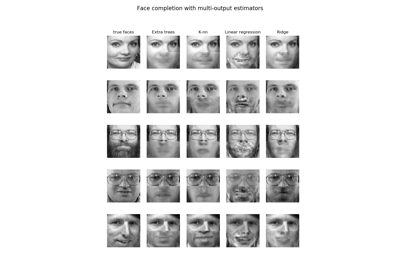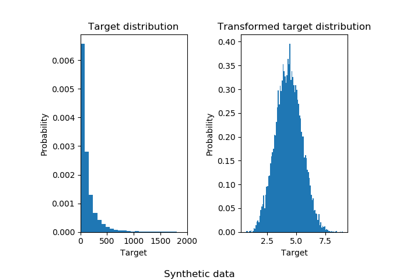# 3.2.4.1.9. sklearn.linear_model.RidgeCV¶

class sklearn.linear_model.RidgeCV(alphas=(0.1, 1.0, 10.0), fit_intercept=True, normalize=False, scoring=None, cv=None, gcv_mode=None, store_cv_values=False)[source]

Ridge regression with built-in cross-validation.

See glossary entry for cross-validation estimator.

By default, it performs Generalized Cross-Validation, which is a form of efficient Leave-One-Out cross-validation.

Read more in the User Guide.

Parameters: alphas : numpy array of shape [n_alphas] Array of alpha values to try. Regularization strength; must be a positive float. Regularization improves the conditioning of the problem and reduces the variance of the estimates. Larger values specify stronger regularization. Alpha corresponds to C^-1 in other linear models such as LogisticRegression or LinearSVC. If using generalized cross-validation, alphas must be positive. fit_intercept : boolean Whether to calculate the intercept for this model. If set to false, no intercept will be used in calculations (e.g. data is expected to be already centered). normalize : boolean, optional, default False This parameter is ignored when fit_intercept is set to False. If True, the regressors X will be normalized before regression by subtracting the mean and dividing by the l2-norm. If you wish to standardize, please use sklearn.preprocessing.StandardScaler before calling fit on an estimator with normalize=False. scoring : string, callable or None, optional, default: None A string (see model evaluation documentation) or a scorer callable object / function with signature scorer(estimator, X, y). If None, the negative mean squared error if cv is ‘auto’ or None (i.e. when using generalized cross-validation), and r2 score otherwise. cv : int, cross-validation generator or an iterable, optional Determines the cross-validation splitting strategy. Possible inputs for cv are: None, to use the efficient Leave-One-Out cross-validation (also known as Generalized Cross-Validation). integer, to specify the number of folds. CV splitter, An iterable yielding (train, test) splits as arrays of indices. For integer/None inputs, if y is binary or multiclass, sklearn.model_selection.StratifiedKFold is used, else, sklearn.model_selection.KFold is used. Refer User Guide for the various cross-validation strategies that can be used here. gcv_mode : {None, ‘auto’, ‘svd’, eigen’}, optional Flag indicating which strategy to use when performing Generalized Cross-Validation. Options are: 'auto' : use 'svd' if n_samples > n_features, otherwise use 'eigen' 'svd' : force use of singular value decomposition of X when X is dense, eigenvalue decomposition of X^T.X when X is sparse. 'eigen' : force computation via eigendecomposition of X.X^T  The ‘auto’ mode is the default and is intended to pick the cheaper option of the two depending on the shape of the training data. store_cv_values : boolean, default=False Flag indicating if the cross-validation values corresponding to each alpha should be stored in the cv_values_ attribute (see below). This flag is only compatible with cv=None (i.e. using Generalized Cross-Validation). cv_values_ : array, shape = [n_samples, n_alphas] or shape = [n_samples, n_targets, n_alphas], optional Cross-validation values for each alpha (if store_cv_values=True and cv=None). After fit() has been called, this attribute will contain the mean squared errors (by default) or the values of the {loss,score}_func function (if provided in the constructor). coef_ : array, shape = [n_features] or [n_targets, n_features] Weight vector(s). intercept_ : float | array, shape = (n_targets,) Independent term in decision function. Set to 0.0 if fit_intercept = False. alpha_ : float Estimated regularization parameter.

Ridge
Ridge regression
RidgeClassifier
Ridge classifier
RidgeClassifierCV
Ridge classifier with built-in cross validation

Examples

>>> from sklearn.datasets import load_diabetes
>>> from sklearn.linear_model import RidgeCV
>>> clf = RidgeCV(alphas=[1e-3, 1e-2, 1e-1, 1]).fit(X, y)
>>> clf.score(X, y)
0.5166...


Methods

 fit(self, X, y[, sample_weight]) Fit Ridge regression model get_params(self[, deep]) Get parameters for this estimator. predict(self, X) Predict using the linear model score(self, X, y[, sample_weight]) Returns the coefficient of determination R^2 of the prediction. set_params(self, \*\*params) Set the parameters of this estimator.
__init__(self, alphas=(0.1, 1.0, 10.0), fit_intercept=True, normalize=False, scoring=None, cv=None, gcv_mode=None, store_cv_values=False)[source]
fit(self, X, y, sample_weight=None)[source]

Fit Ridge regression model

Parameters: X : array-like, shape = [n_samples, n_features] Training data. If using GCV, will be cast to float64 if necessary. y : array-like, shape = [n_samples] or [n_samples, n_targets] Target values. Will be cast to X’s dtype if necessary sample_weight : float or array-like of shape [n_samples] Sample weight self : object

Notes

When sample_weight is provided, the selected hyperparameter may depend on whether we use generalized cross-validation (cv=None or cv=’auto’) or another form of cross-validation, because only generalized cross-validation takes the sample weights into account when computing the validation score.

get_params(self, deep=True)[source]

Get parameters for this estimator.

Parameters: deep : boolean, optional If True, will return the parameters for this estimator and contained subobjects that are estimators. params : mapping of string to any Parameter names mapped to their values.
predict(self, X)[source]

Predict using the linear model

Parameters: X : array_like or sparse matrix, shape (n_samples, n_features) Samples. C : array, shape (n_samples,) Returns predicted values.
score(self, X, y, sample_weight=None)[source]

Returns the coefficient of determination R^2 of the prediction.

The coefficient R^2 is defined as (1 - u/v), where u is the residual sum of squares ((y_true - y_pred) ** 2).sum() and v is the total sum of squares ((y_true - y_true.mean()) ** 2).sum(). The best possible score is 1.0 and it can be negative (because the model can be arbitrarily worse). A constant model that always predicts the expected value of y, disregarding the input features, would get a R^2 score of 0.0.

Parameters: X : array-like, shape = (n_samples, n_features) Test samples. For some estimators this may be a precomputed kernel matrix instead, shape = (n_samples, n_samples_fitted], where n_samples_fitted is the number of samples used in the fitting for the estimator. y : array-like, shape = (n_samples) or (n_samples, n_outputs) True values for X. sample_weight : array-like, shape = [n_samples], optional Sample weights. score : float R^2 of self.predict(X) wrt. y.

Notes

The R2 score used when calling score on a regressor will use multioutput='uniform_average' from version 0.23 to keep consistent with metrics.r2_score. This will influence the score method of all the multioutput regressors (except for multioutput.MultiOutputRegressor). To specify the default value manually and avoid the warning, please either call metrics.r2_score directly or make a custom scorer with metrics.make_scorer (the built-in scorer 'r2' uses multioutput='uniform_average').

set_params(self, **params)[source]

Set the parameters of this estimator.

The method works on simple estimators as well as on nested objects (such as pipelines). The latter have parameters of the form <component>__<parameter> so that it’s possible to update each component of a nested object.

Returns: self

## 3.2.4.1.9.1. Examples using sklearn.linear_model.RidgeCV¶Face completion with a multi-output estimatorsEffect of transforming the targets in regression model